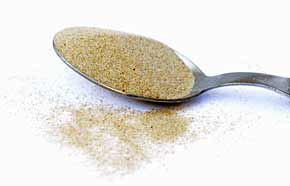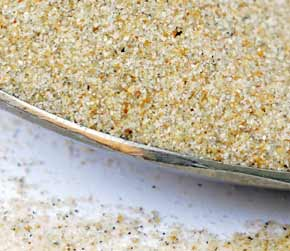﻿ Beach Sand 1 cup US volume to cubic meters converter

beach sand conversion

Amount: 1 cup US (cup us) of volume Equals: 0.00024 cubic meters (m3) in volume

Converting cup US to cubic meters value in the beach sand units scale.

TOGGLE :   from cubic meters into cups US in the other way around.

beach sand from cup US to cubic meter Conversion Results:

Enter a New cup US Amount of beach sand to Convert From

* Whole numbers, decimals or fractions (ie: 6, 5.33, 17 3/8)
* Precision is how many numbers after decimal point (1 - 9)

Enter Amount :
Decimal Precision :

CONVERT :   between other beach sand measuring units - complete list.

Conversion calculator for webmasters.

Beach sand weight vs. volume units

Beach sand has quite high density, it's heavy and it easily leaks into even tiny gaps or other opened spaces. No wonder it absorbs and conducts heat energy from the sun so well. However, this sand does not have the heat conductivity as high as glass does, or fireclay and firebricks, or dense concrete. A fine beach sand in dry form was used for taking these measurements.Convert beach sand measuring units between cup US (cup us) and cubic meters (m3) but in the other reverse direction from cubic meters into cups US.

 conversion result for beach sand: From Symbol Equals Result To Symbol 1 cup US cup us = 0.00024 cubic meters m3

Converter type: beach sand measurements

This online beach sand from cup us into m3 converter is a handy tool not just for certified or experienced professionals.

First unit: cup US (cup us) is used for measuring volume.
Second: cubic meter (m3) is unit of volume.

beach sand per 0.00024 m3 is equivalent to 1 what?

The cubic meters amount 0.00024 m3 converts into 1 cup us, one cup US. It is the EQUAL beach sand volume value of 1 cup US but in the cubic meters volume unit alternative.

How to convert 2 cups US (cup us) of beach sand into cubic meters (m3)? Is there a calculation formula?

First divide the two units variables. Then multiply the result by 2 - for example:
0.0002365882365 * 2 (or divide it by / 0.5)

QUESTION:
1 cup us of beach sand = ? m3

1 cup us = 0.00024 m3 of beach sand

Other applications for beach sand units calculator ...

With the above mentioned two-units calculating service it provides, this beach sand converter proved to be useful also as an online tool for:
1. practicing cups US and cubic meters of beach sand ( cup us vs. m3 ) measuring values exchange.
2. beach sand amounts conversion factors - between numerous unit pairs variations.
3. working with mass density - how heavy is a volume of beach sand - values and properties.

International unit symbols for these two beach sand measurements are:

Abbreviation or prefix ( abbr. short brevis ), unit symbol, for cup US is:
cup us
Abbreviation or prefix ( abbr. ) brevis - short unit symbol for cubic meter is:
m3

One cup US of beach sand converted to cubic meter equals to 0.00024 m3

How many cubic meters of beach sand are in 1 cup US? The answer is: The change of 1 cup us ( cup US ) volume unit of beach sand measure equals = to volume 0.00024 m3 ( cubic meter ) as the equivalent measure within the same beach sand substance type.

In principle with any measuring task, switched on professional people always ensure, and their success depends on, they get the most precise conversion results everywhere and every-time. Not only whenever possible, it's always so. Often having only a good idea ( or more ideas ) might not be perfect nor good enough solution. If there is an exact known measure in cup us - cups US for beach sand amount, the rule is that the cup US number gets converted into m3 - cubic meters or any other beach sand unit absolutely exactly.

Conversion for how many cubic meters ( m3 ) of beach sand are contained in a cup US ( 1 cup us ). Or, how much in cubic meters of beach sand is in 1 cup US? To link to this beach sand cup US to cubic meters online converter simply cut and paste the following.
The link to this tool will appear as: beach sand from cup US (cup us) to cubic meters (m3) conversion.

I've done my best to build this site for you- Please send feedback to let me know how you enjoyed visiting.